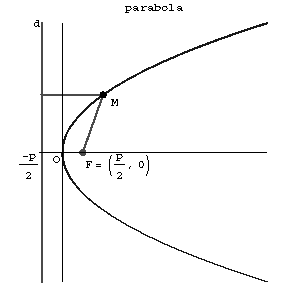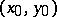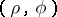# Parabola

A plane curve obtained as the intersection of a circular cone with a plane not passing through the vertex of the cone and parallel to one of its tangent planes. A parabola is a set of pointsin the plane for each of which the distance to a given point(the focus of the parabola) is equal to the distance to a certain given line(the directrix). Thus, a parabola is a conic with eccentricity one. The distancefrom the focus of the parabola to the directrix is called the parameter. A parabola is a symmetric curve; the point of intersection of a parabola with its axis of symmetry is called the vertex of the parabola, the axis of symmetry is called the axis of the parabola. A diameter of a parabola is any straight line parallel to its axis, and can be defined as the locus of the midpoints of a set of parallel chords.Figure: p071150a

A parabola is a non-central second-order curve. Its canonical equation has the formThe equation of the tangent to a parabola at the pointisThe equation of a parabola in polar coordinatesisA parabola has an optical property: Light rays emanating from the focus travel, after reflection in the parabola, parallel to the axis.